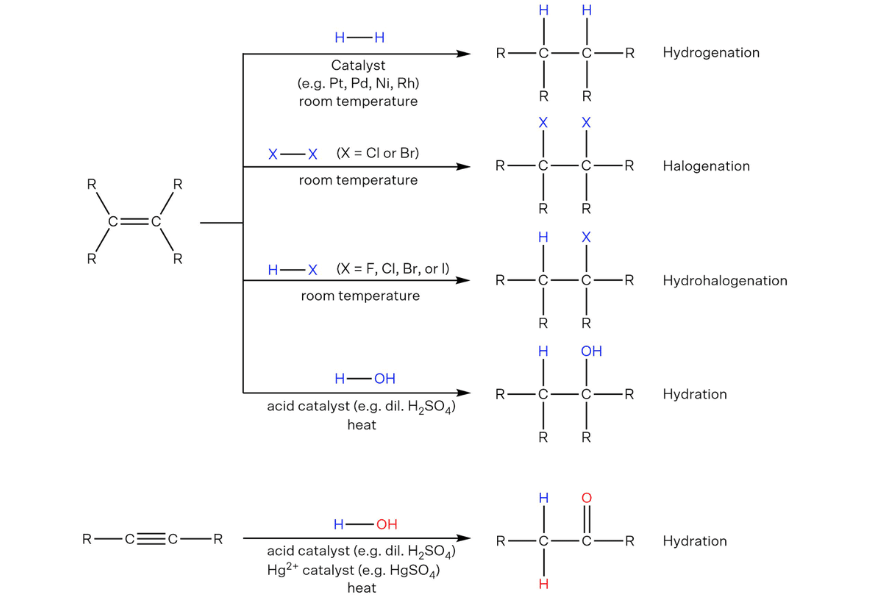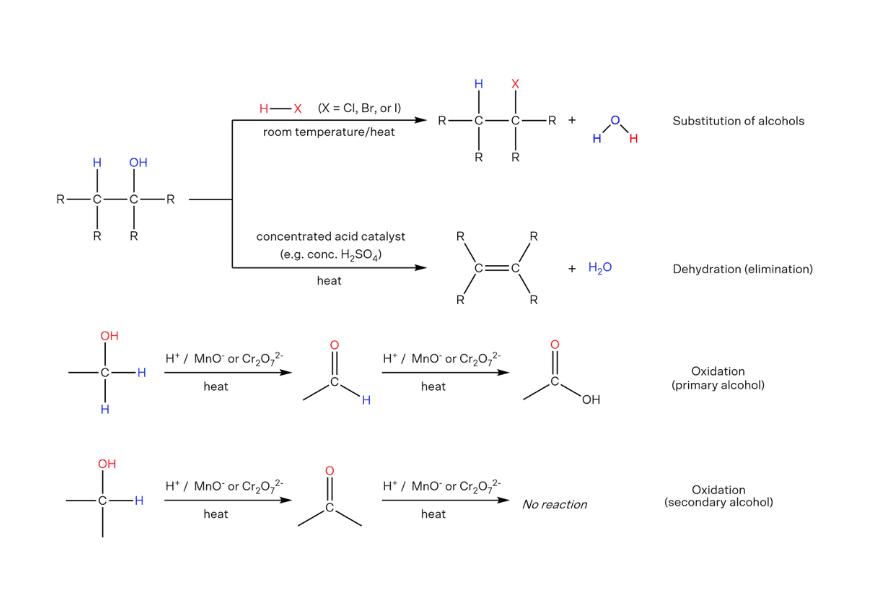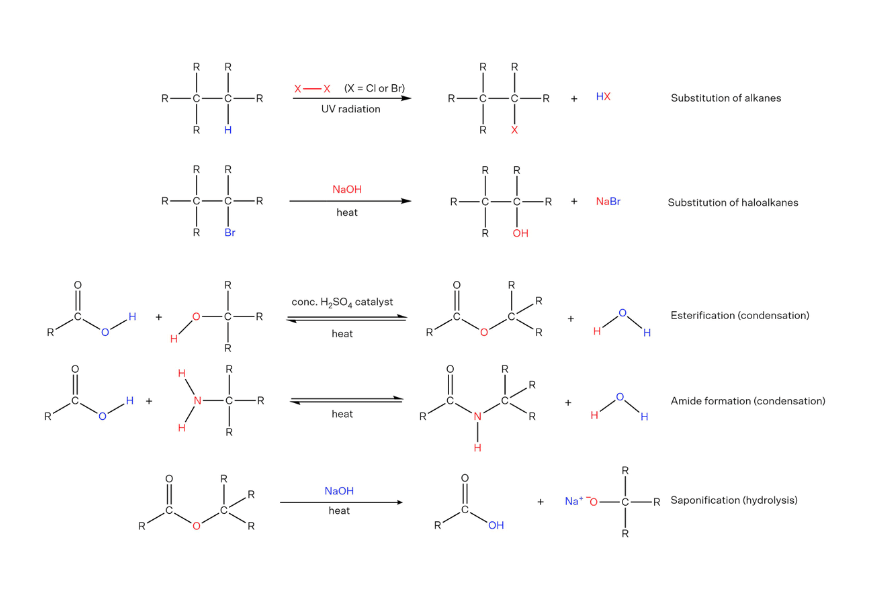# List Of Important Chemical Reactions Year 12 Students Must Know

Do you find yourself flipping through pages of notes just to find a particular chemical reaction? Well, you don’t have to do that anymore! Here are all the important chemical reactions Year 12 students must know!

1. Module 5: Equilibrium and Acid Reactions
2. Module 6: Acid/Base Reactions
3. Module 7: Organic Chemistry

## Module 5: Equilibrium and Acid Reactions

### Combustion of metals

Word equation: metal + oxygen → metal oxide

Examples:

Burning magnesium: $$2Mg_{(s)} \ + \ O_{2(g)} \ → \ 2M_{g}O_{(s)}$$

Burning steel wool: $$4Fe_{(s)} \ + \ 3O_{2(g)} \ → \ 2Fe_{2}O_{3(s)}$$

### Combustion of hydrocarbons and alcohols

1. Complete combustion produces carbon dioxide and water.

Examples:

Hydrocarbon: $$C_{3}H_{8(g)} \ + \ 5O_{2(g)} \ → \ 3CO_{2(g)} \ + \ 4H_{2}O_{(l)}$$

Alcohol: $$C_{2}H_{5}OH_{(l)} \ + \ 3O_{2(g)} \ → \ 2CO_{2(g)} \ + \ 3H_{2}O_{(l)}$$

2. Incomplete combustion occurs when insufficient oxygen is present for a complete reaction to occur.

Water and carbon monoxide ($$CO_{(g)}$$) and/or soot ($$C_{(s)}$$) are produced in an incomplete combustion.

### Photosynthesis

Word equation: carbon dioxide + water → glucose + oxygen

Chemical equation: $$6CO_{2(g)} \ + \ 6H_{2}O_{(l)} \ → \ C_{6}H_{12}O_{6(s)} \ + \ 6O_{2(g)}$$

### Cobalt chloride hydrate equilibrium

$$[CoCl_{4}]^{2-} \ _{(aq)} \ (blue) \ + \ 6H_{2}O_{(l)} \ ⇌ \ [Co(H_{2}O)_{6}]^{2+} \ _{(aq)} \ (pink) \ + \ 4Cl^{-} \ _{(aq)}$$

### Iron thiocyanate equilibrium

$$Fe^{3+} \ _{(aq)} \ \text{(pale yellow)} \ + \ SCN^{-} \ _{(aq)} \ ⇌ \ [FeSCN]^{2+} \ _{(aq)} \ \text{(deep red)}$$

### Nitrogen dioxide equilibrium

$$2NO_{2(g)} \ (brown) \ ⇌ \ N_{2}O_{4(g)} \ \text{(colourless)}$$

### Dissolution equilibrium

A saturated solution of an ionic compound will be in dynamic equilibrium.

Example: $$CuSO_{4(aq)} \ ⇌ \ Cu^{2+} \ _(aq) \ + \ SO_{4^{2-} (aq) }$$

### Precipitation reactions

A good knowledge of the solubility rules is essential to determining whether a precipitation reaction will occur when solutions containing ionic compounds are mixed.

Example:

Full formula equation: $$BaCl_{2(aq)} \ + \ Na_{2}SO_{4(aq)} \ → \ BaSO_{4(s)} \ + \ 2NaCl_{(aq)}$$

Net ionic equation: $$Ba^{2+} \ _{(aq)} \ + \ SO_{4^{2-} (aq)} \ → \ BaSO_{4(s)}$$

## Module 6: Acid/Base Reactions

### Ionisation

1. Strong acids undergo complete ionisation in water

Example: $$HCl_{(aq)} \ + \ H_{2}O_{(l)} \ → \ H_{3}O^{+} \ _{(aq)} \ + \ Cl^{-} \ _{(aq)}$$

$$(HCl_{(aq)} \ → \ H^{+} \ _{(aq)} \ + \ Cl^{-} \ _{(aq)})$$

2. Weak acids undergo partial ionisation in water

Example:  $$CH_{3}COOH_{(aq)} \ + \ H_{2}O_{(l)} \ ⇌ \ H_{3}O^{+} \ _{(aq)} \ + \ CH_{3}COO^{-} \ _{(aq)}$$

$$(CH_{3}COOH_{(aq)} \ ⇌ \ H^{+} \ _{(aq)} \ + \ CH_{3}COO^{-} \ _{(aq)})$$

3. Strong bases undergo complete dissociation in water

Example: $$NaOH_{(aq)} \ → \ Na^{+} \ _{(aq)} \ + \ OH^{-} \ _{(aq)}$$

4. Weak bases undergo partial ionisation in water

Example: $$NH_{3(aq)} \ + \ H_{2}O_{(l)} \ ⇌ \ NH_{4^{+} (aq)} \ + \ OH^{-} \ _{(aq)}$$

### Neutralisation

Word equation: acid + base → salt + water

Example: $$H_{2} \color{red}{SO_{4}} _{(aq)} \ + \ 2 \color{blue}{K} OH_{(aq)} \ → \ \color{blue}{K_{2}} \color{red}{SO_{4}} _{(aq)} \ + \ H_{2}O_{(l)}$$

### Acids and carbonates

Word equation: acid + carbonate → salt + water + carbon dioxide

Example: $$2H \color{red}{NO_{3}} _{(aq)} \ + \ \color{blue}{Na} _{2} CO_{3(s)} \ → \ 2 \color{blue}{Na} \color{red}{NO_{3}} _{(aq)} \ + \ H_{2}O_{(l)} \ + \ CO_{2(g)}$$

### Acids and metals

Word equation: acid + metal → salt + hydrogen gas

Example: $$2H \color{red}{Cl} _{(aq)} \ + \ \color{blue}{Mg} _{(s)} \ → \ \color{blue}{Mg} \color{red}{Cl} _{2(aq)} \ + \ H_{2(g)}$$

### Acid and ammonia:

Word equation: acid + ammonia → ammonium salt

Example: $$HCl_{(aq)} \ + \ NH_{3(aq)} \ → \ NH_{4}Cl_{(aq)}$$

## Module 7: Organic Chemistry

### Addition reactions of unsaturated hydrocarbons### Reactions of alcohols### Combustion of hydrocarbons and alcohols

1. Complete combustion produces carbon dioxide and water.

Examples:

Hydrocarbon: $$C_{3}H_{8(g)} \ + \ 5O_{2(g)} \ → \ 3CO_{2(g)} \ + \ 4H_{2}O_{(l)}$$

Alcohol: $$C_{2}H_{5}OH_{(l)} \ + \ 3O_{2(g)} \ → \ 2CO_{2(g)} \ + \ 3H_{2}O_{(l)}$$

2. Incomplete combustion occurs when insufficient oxygen is present for a complete reaction to occur.

Water and carbon monoxide $$(CO_{(g)})$$ and/or soot $$(C_{(s)})$$ are produced in an incomplete combustion.

### Fermentation

Fermentation of glucose: $$C_{6}H_{12}O_{6(aq)} \ → \ 2C_{2}H_{5}OH_{(l)} \ + \ 2CO_{2(g)}$$

Conditions for fermentation:

• Zymase (enzyme in yeast)
• Aqueous solution of sugar
• Anaerobic condition
• Typically 30–40 °C, but depends on yeast strain

## Additional reactions that are good to know

### Redox

1. Oxidation: $$M_{(s)} \ → \ M^{+} \ _{(aq)} \ + \ e^{-}$$

2. Reduction: $$M^{+} \ _{(aq)} \ + \ e^{-} \ → \ M_{(s)}$$

3. Redox equation: Combine oxidation and reduction equations and balance electrons.

Example

• Oxidation: $$Cu(s) → Cu2+(aq) + 2e-$$
• Reduction:  $$Ag+(aq) + e- → Ag(s)$$
• $$1:2$$ for $$Cu:Ag$$, so combine with 2x reduction equation
• $$Cu_{(s)} \ + \ 2Ag^{+} \ _{(aq)} \ + \ 2e^{-} \ → \ Cu^{2+} \ _{(aq)} \ + \ 2e^{-} \ + \ 2Ag_{(s)}$$
• Cancel the electrons: $$Cu_{(s)} \ + \ 2Ag^{+} _{(aq)} \ → \ Cu^{2+} \ _{(aq)} \ + \ 2Ag_{(s)}$$

4. Limewater test:  $$Ca(OH)_{2(aq)} \ + \ CO_{2(g)} \ → \ CaCO_{3(s)} \ + \ H_{2}O_{(l)}$$

,

#### Related Posts

Platform Slot Gacor CMS Checker